# SAT Math Multiple Choice Question 586: Answer and Explanation

### Test Information

Question: 586

1.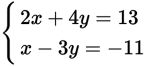Based on the system of equations above, what is the value of the sum of x and y?

• A.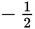• B. 3
• C.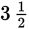• D. 4

Explanation:

B

Difficulty: Medium

Category: Heart of Algebra / Systems of Linear Equations

Strategic Advice: Solve the system of equations using substitution. Then, check that you answered the right question (find the sum of x and y).

Getting to the Answer: First, solve the second equation for x to get x = 3y – 11, and then substitute this equation into the first equation to find y: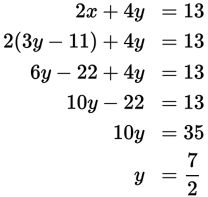Now, substitute the result into x = 3y – 11 and simplify to find x: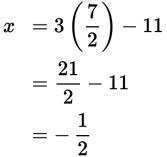The question asks for the sum, so add x and y to get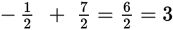.